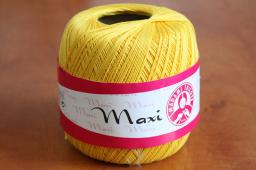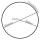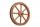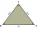# Circle r,D

Calculate the diameter and radius of the circle if it has length 26.59 cm.

Correct result:

r =  4.2319 cm
D =  8.4639 cm

#### Solution:We would be pleased if you find an error in the word problem, spelling mistakes, or inaccuracies and send it to us. Thank you!#### You need to know the following knowledge to solve this word math problem:

We encourage you to watch this tutorial video on this math problem:

## Next similar math problems:

• CircleWhat is the radius of the circle whose perimeter is 6 cm?
• Circumference - a simpleWhat is the ratio of the circumference of any circle and its diameter? Write the result as a real number rounded to 2 decimal places.
• Two circlesTwo circles with a radius 4 cm and 3 cm have a center distance 0.5cm. How many common points have these circles?
• Value of expressionX=2, y=-5 and z=3 what is the value of x-2y?
• Circle - simpleCalculate the area of a circle in dm2, if its circumference is 31.4 cm.
• Wheel diameterA 1m diameter wheel rolled along a 100m long track. How many times did it turn?
• WellRope with a bucket is fixed on the shaft with the wheel. The shaft has a diameter 50 cm. How many meters will drop bucket when the wheels turn 15 times?
• Sides of triangleTriangle circumference with two identical sides is 117cm. The third side measures 44cm. How many cms do you measure one of the same sides?
• FlowerbedIn the park there is a large circular flowerbed with a diameter of 12 m. Jakub circulated him ten times and the smaller Vojtoseven times. How many meters each went by and how many meters did Jakub run more than Vojta?
• Clock handsThe second hand has a length of 1.5 cm. How long does the endpoint of this hand travel in one day?
• Simplify 2Simplify expression: 5ab-7+3ba-9
• MineWheel in traction tower has a diameter 4 m. How many meters will perform an elevator cabin if wheel rotates in the same direction 89 times?
• Circle - simpleThe circumference of a circle is 198 mm. How long in mm is its diameter?
• Find theFind the radius of the circular base of the vessel, whose perimeter is 2.51 m. Write the result to one decimal place in meters. Via the π sign - Ludolph's number
• Area to perimeterCalculate circle circumference if its area is 254.34cm2
• ParallelogramCalculate the missing side of a parallelogram, if you know the perimeter o and one side: a) o = 7.2 cm; b = 1.8 cm b) o = 5.4 cm; a = 1.9 cm
• Four circles1) Calculate the circle radius if its area is 400 cm square 2) Calculate the radius of the circle whose circumference is 400 cm. 3) Calculate circle circumference if its area is 400 cm square 4) Calculate the circle's area if perimeter 400 cm.